Generative Adversarial Networks Library: pygan¶

pygan is Python library to implement Generative Adversarial Networks(GANs), Conditional GANs, Adversarial Auto-Encoders(AAEs), and Energy-based Generative Adversarial Network(EBGAN).

This library makes it possible to design the Generative models based on the Statistical machine learning problems in relation to Generative Adversarial Networks(GANs), Conditional GANs, Adversarial Auto-Encoders(AAEs), and Energy-based Generative Adversarial Network(EBGAN) to practice algorithm design for semi-supervised learning. But this library provides components for designers, not for end-users of state-of-the-art black boxes. Briefly speaking the philosophy of this library, give user hype-driven blackboxes and you feed him for a day; show him how to design algorithms and you feed him for a lifetime. So algorithm is power.

Installation¶

Install using pip:

pip install pygan

Source code¶

The source code is currently hosted on GitHub.

Python package index(PyPI)¶

Installers for the latest released version are available at the Python package index.

Dependencies¶

• numpy: v1.13.3 or higher.

Option¶

• pydbm: v1.4.9 or higher.
• Only if you want to implement the components based on this library.

Documentation¶

Full documentation is available on https://code.accel-brain.com/Generative-Adversarial-Networks/ . This document contains information on functionally reusability, functional scalability and functional extensibility.

Description¶

pygan is Python library to implement Generative Adversarial Networks(GANs), Conditional GANs, Adversarial Auto-Encoders(AAEs), and Energy-based Generative Adversarial Network(EBGAN).

The Generative Adversarial Networks(GANs) (Goodfellow et al., 2014) framework establishes a min-max adversarial game between two neural networks – a generative model, G, and a discriminative model, D. The discriminator model, D(x), is a neural network that computes the probability that a observed data point x in data space is a sample from the data distribution (positive samples) that we are trying to model, rather than a sample from our generative model (negative samples). Concurrently, the generator uses a function G(z) that maps samples z from the prior p(z) to the data space. G(z) is trained to maximally confuse the discriminator into believing that samples it generates come from the data distribution. The generator is trained by leveraging the gradient of D(x) w.r.t. x, and using that to modify its parameters.

Structural extension for Conditional GANs (or cGANs).¶

The Conditional GANs (or cGANs) is a simple extension of the basic GAN model which allows the model to condition on external information. This makes it possible to engage the learned generative model in different “modes” by providing it with different contextual information (Gauthier, J. 2014).

This model can be constructed by simply feeding the data, y, to condition on to both the generator and discriminator. In an unconditioned generative model, because the maps samples z from the prior p(z) are drawn from uniform or normal distribution, there is no control on modes of the data being generated. On the other hand, it is possible to direct the data generation process by conditioning the model on additional information (Mirza, M., & Osindero, S. 2014).

Structural extension for Adversarial Auto-Encoders(AAEs).¶

This library also provides the Adversarial Auto-Encoders(AAEs), which is a probabilistic Auto-Encoder that uses GANs to perform variational inference by matching the aggregated posterior of the feature points in hidden layer of the Auto-Encoder with an arbitrary prior distribution(Makhzani, A., et al., 2015). Matching the aggregated posterior to the prior ensures that generating from any part of prior space results in meaningful samples. As a result, the decoder of the Adversarial Auto-Encoder learns a deep generative model that maps the imposed prior to the data distribution.

Structural extension for Energy-based Generative Adversarial Network(EBGAN).¶

Reusing the Auto-Encoders, this library introduces the Energy-based Generative Adversarial Network (EBGAN) model(Zhao, J., et al., 2016) which views the discriminator as an energy function that attributes low energies to the regions near the data manifold and higher energies to other regions. THe Auto-Encoders have traditionally been used to represent energy-based models. When trained with some regularization terms, the Auto-Encoders have the ability to learn an energy manifold without supervision or negative examples. This means that even when an energy-based Auto-Encoding model is trained to reconstruct a real sample, the model contributes to discovering the data manifold by itself.

The Commonality/Variability Analysis in order to practice object-oriented design.¶

From perspective of commonality/variability analysis in order to practice object-oriented design, the concepts of GANs and AAEs can be organized as follows: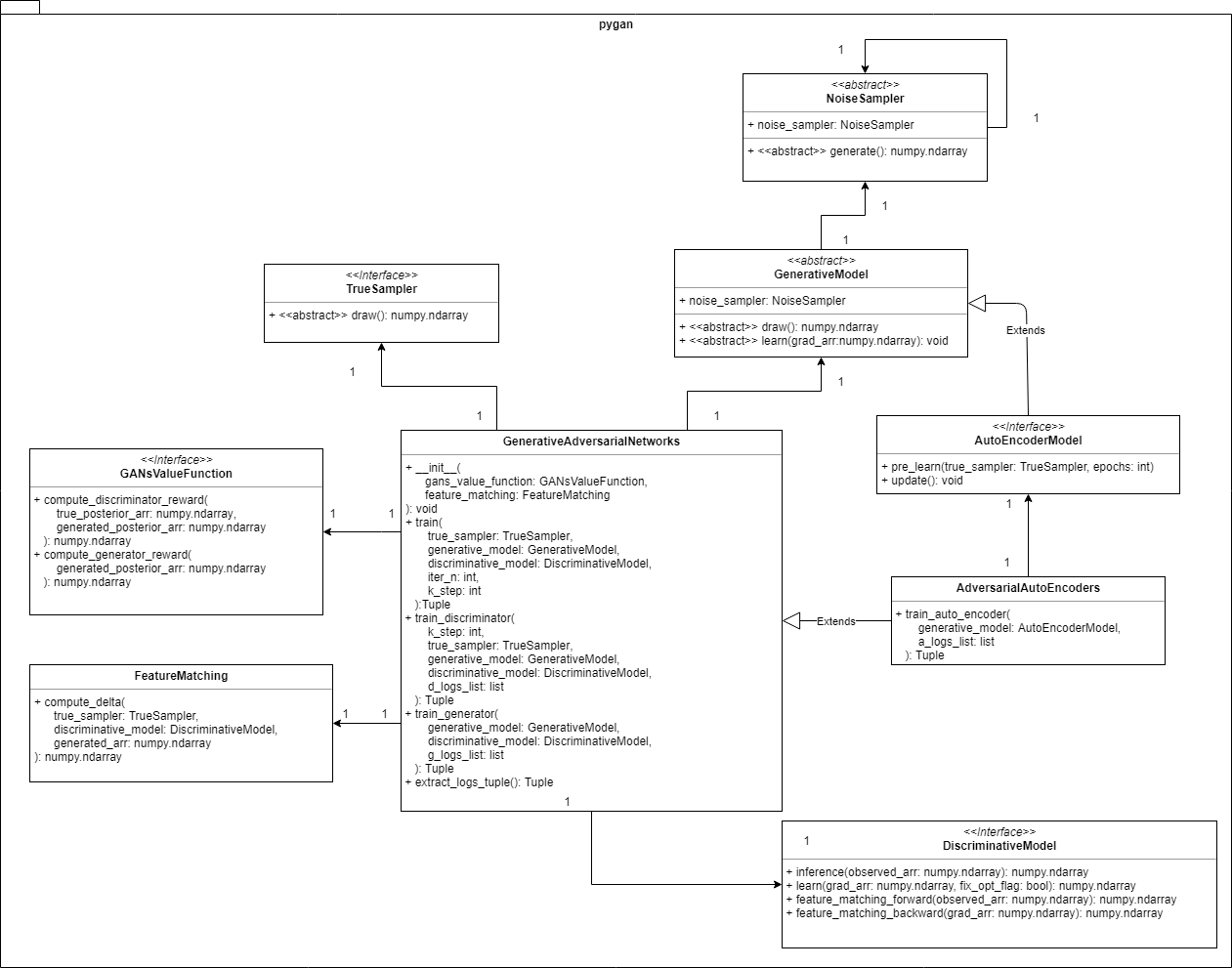The configuration is based on the Strategy Pattern, which provides a way to define a family of algorithms implemented by inheriting the interface or abstract class such as TrueSampler, NoiseSampler, GenerativeModel, and DiscriminativeModel, where … - TrueSampler is the interface to provide x by drawing from the true distributions. - GenerativeModel is the abstract class to generate G(z) by drawing from the fake distributions with NoiseSampler which provides z. - DiscriminativeModel is the interface to inference that observed data points are true or fake as a D(x).

This pattern is encapsulate each one as an object, and make them interchangeable from the point of view of functionally equivalent. This library provides sub classes such as Neural Networks, Convolutional Neural Networks, and LSTM networks. Althogh those models are variable from the view points of learning algorithms, but as a GenerativeModel or a DiscriminativeModel those models have common function.

GenerativeAdversarialNetworks is a Context in the Strategy Pattern, controlling the objects of TrueSampler, GenerativeModel, and DiscriminativeModel in order to train G(z) and D(x). This context class also calls the object of GANsValueFunction, whose function is computing the rewards or gradients in GANs framework.

The structural extension from GANs to AAEs is achieved by the inheritance of two classes: GenerativeModel and GenerativeAdversarialNetworks. One of the main concepts of AAEs, which is worthy of special mention, can be considered that the Auto-Encoders can be transformed into the generative Models. Therefore this library firstly implements a AutoEncoderModel by inheriting GenerativeModel. Next, this library watches closely that the difference between GANs and AAEs brings us different context in the Strategy Pattern in relation to the learning algorithm of Auto-Encoders. By the addition of the AutoEncoderModel’s learning method, this library provieds AdversarialAutoEncoders which is-a GenerativeAdversarialNetworks and makes it possible to train not only GenerativeModel and DiscriminativeModel but also AutoEncoderModel.

Furthermore, FeatureMatching is a value function with so-called Feature matching technic, which addresses the instability of GANs by specifying a new objective for the generator that prevents it from overtraining on the current discriminator(Salimans, T., et al., 2016).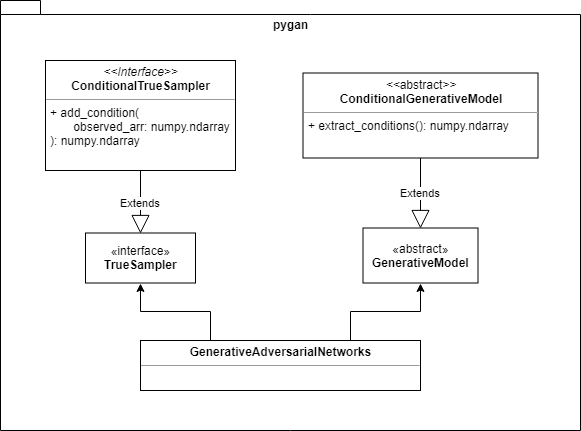Like Yang, L. C., et al. (2017), this library implements the Conditioner to conditon on external information. As class configuration in this library, the Conditioner is divided into two, ConditionalGenerativeModel and ConditionalTrueSampler. This library consider that the ConditionalGenerativeModel and ConditionalTrueSampler contain Conditioner of the Conditional GANs to reduce the burden of architectural design. The controller GenerativeAdversarialNetworks functionally uses the conditions in a black boxed state.

Usecase: Generating Sine Waves by GANs.¶

Set hyperparameters.

# Batch size
batch_size = 20
# The length of sequences.
seq_len = 30
# The dimension of observed or feature points.
dim = 5

Import Python modules.

# is-a `TrueSampler`.
from pygan.truesampler.sine_wave_true_sampler import SineWaveTrueSampler
# is-a `NoiseSampler`.
from pygan.noisesampler.uniform_noise_sampler import UniformNoiseSampler
# is-a `GenerativeModel`.
from pygan.generativemodel.lstm_model import LSTMModel
# is-a `DiscriminativeModel`.
from pygan.discriminativemodel.nn_model import NNModel
# is-a `GANsValueFunction`.
from pygan.gansvaluefunction.mini_max import MiniMax
# GANs framework.

Setup TrueSampler.

true_sampler = SineWaveTrueSampler(
batch_size=batch_size,
seq_len=seq_len,
dim=dim
)

Setup NoiseSampler and GenerativeModel.

noise_sampler = UniformNoiseSampler(
# Lower boundary of the output interval.
low=-1,
# Upper boundary of the output interval.
high=1,
# Output shape.
output_shape=(batch_size, 1, dim)
)

generative_model = LSTMModel(
batch_size=batch_size,
seq_len=seq_len,
input_neuron_count=dim,
hidden_neuron_count=dim
)
generative_model.noise_sampler = noise_sampler

Setup DiscriminativeModel with pydbm library.

# Computation graph for Neural network.
from pydbm.synapse.nn_graph import NNGraph
# Layer object of Neural network.
from pydbm.nn.nn_layer import NNLayer
#\$ Logistic function or Sigmoid function which is-a `ActivatingFunctionInterface`.
from pydbm.activation.logistic_function import LogisticFunction

nn_layer = NNLayer(
graph=NNGraph(
activation_function=LogisticFunction(),
# The number of units in hidden layer.
hidden_neuron_count=seq_len * dim,
# The number of units in output layer.
output_neuron_count=1
)
)

discriminative_model = NNModel(
# `list` of `NNLayer`.
nn_layer_list=[nn_layer],
batch_size=batch_size
)

Setup the value function.

gans_value_function = MiniMax()

Setup GANs framework.

gans_value_function=gans_value_function
)

If you want to setup GNAs framework with so-called feature matching technic, which is effective in situations where regular GAN becomes unstable(Salimans, T., et al., 2016), setup GANs framework as follows:

gans_value_function=gans_value_function,
feature_matching=FeatureMatching(
# Weight for results of standard feature matching.
lambda1=0.01,
# Weight for results of difference between generated data points and true samples.
lambda2=0.99
)
)

where lambda1 and lambda2 are trade-off parameters. lambda1 means a weight for results of standard feature matching and lambda2 means a weight for results of difference between generated data points and true samples(Yang, L. C., et al., 2017).

Start training.

generative_model, discriminative_model = GAN.train(
true_sampler,
generative_model,
discriminative_model,
# The number of training iterations.
iter_n=100,
# The number of learning of the discriminative_model.
k_step=10
)

Visualization.¶

Check the rewards or losses.

d_logs_list, g_logs_list = GAN.extract_logs_tuple()

d_logs_list is a list of probabilities inferenced by the discriminator (mean) in the discriminator’s update turn and g_logs_list is a list of probabilities inferenced by the discriminator (mean) in the generator’s update turn.

Visualize the values of d_logs_list.

import matplotlib.pyplot as plt
import seaborn as sns
%config InlineBackend.figure_format = "retina"
plt.style.use("fivethirtyeight")
plt.figure(figsize=(20, 10))
plt.plot(d_logs_list)
plt.xlabel("Epochs")
plt.ylabel("Loss")
plt.show()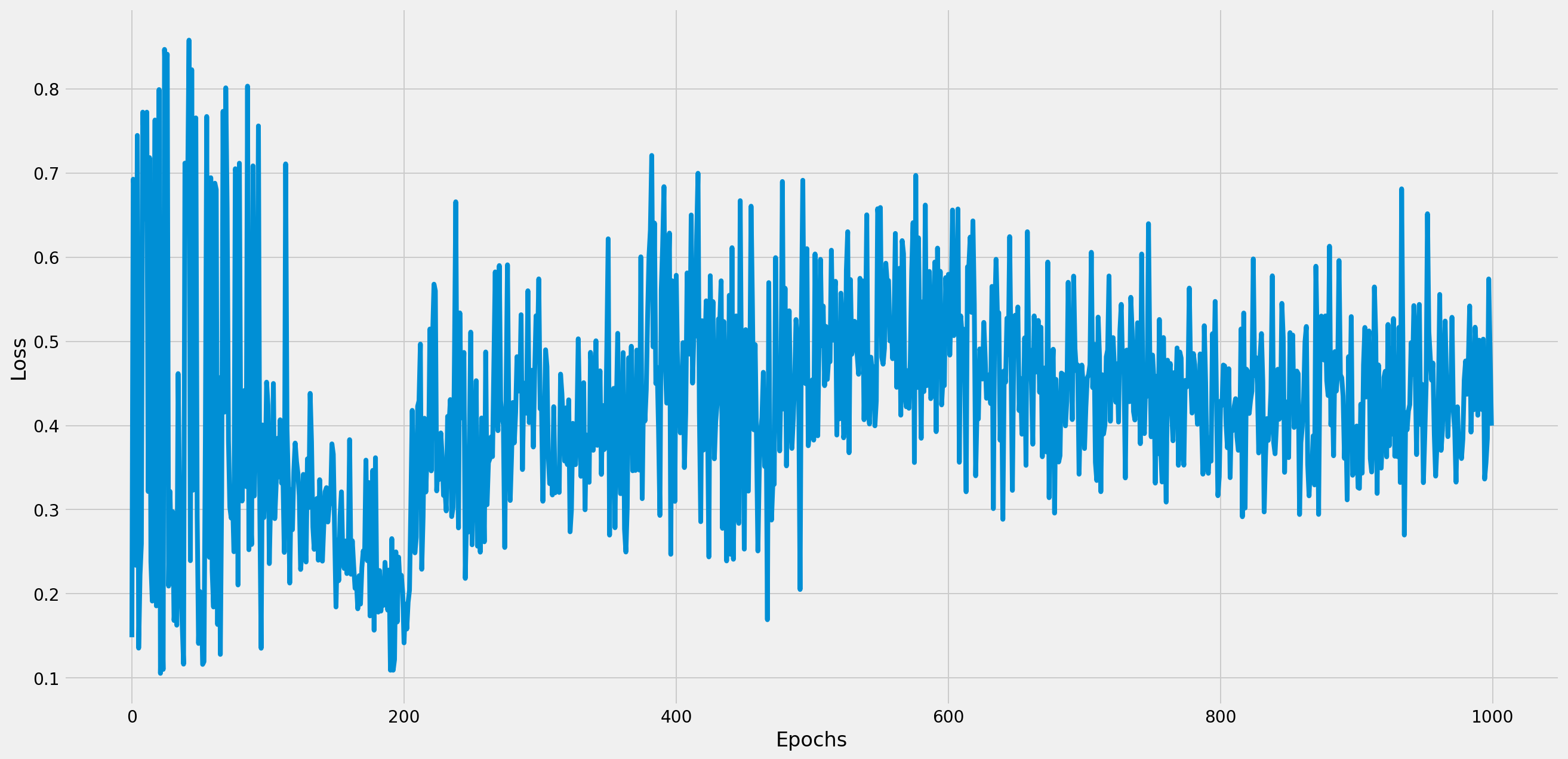Similarly, visualize the values of g_logs_list.

plt.figure(figsize=(20, 10))
plt.plot(g_logs_list)
plt.xlabel("Epochs")
plt.ylabel("Loss")
plt.show()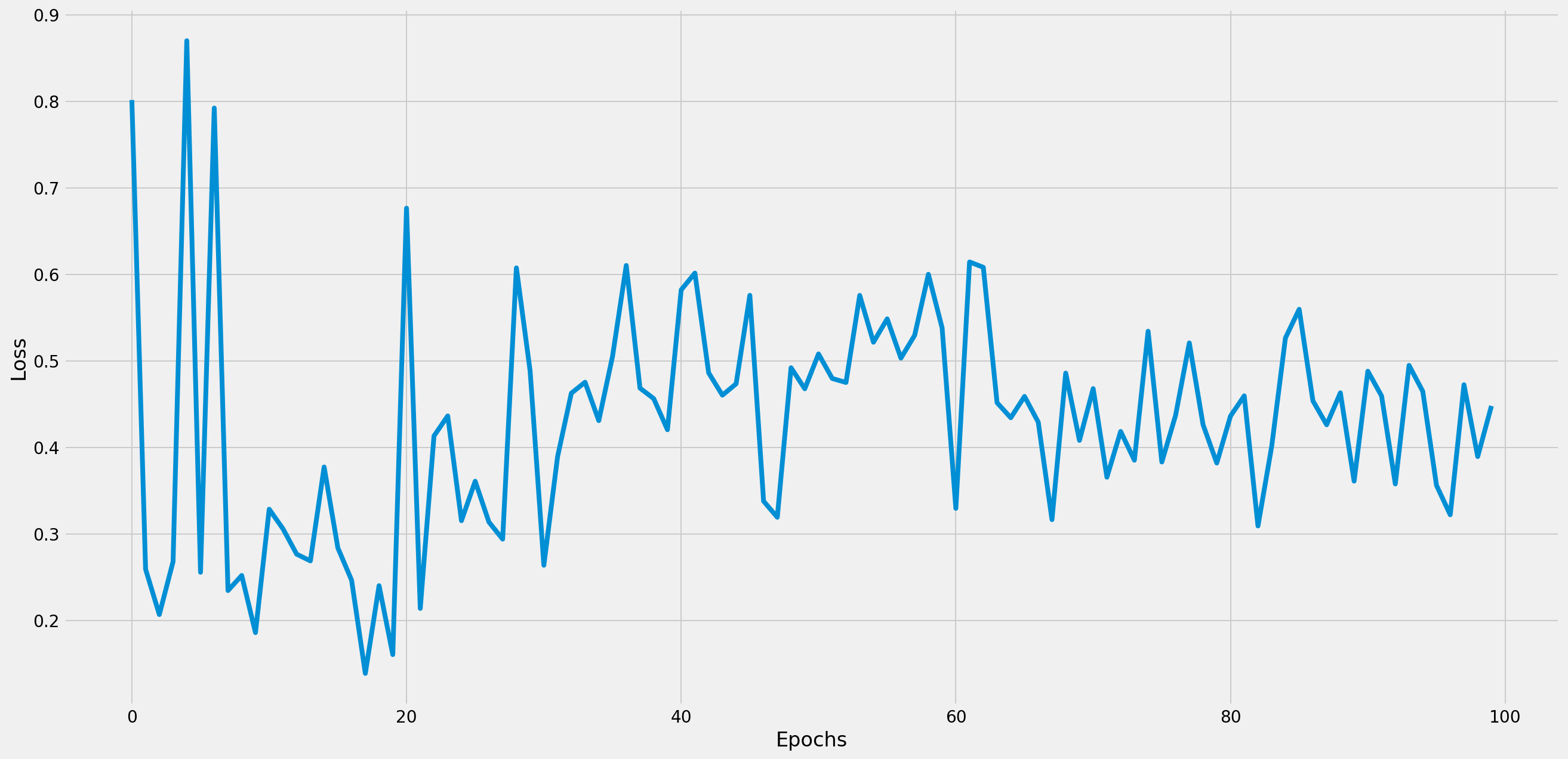As the training progresses, the values are close to 0.5.

Generation.¶

Plot a true distribution and generated data points to check how the discriminator was confused by the generator.

true_arr = true_sampler.draw()

plt.style.use("fivethirtyeight")
plt.figure(figsize=(20, 10))
plt.plot(true_arr)
plt.show()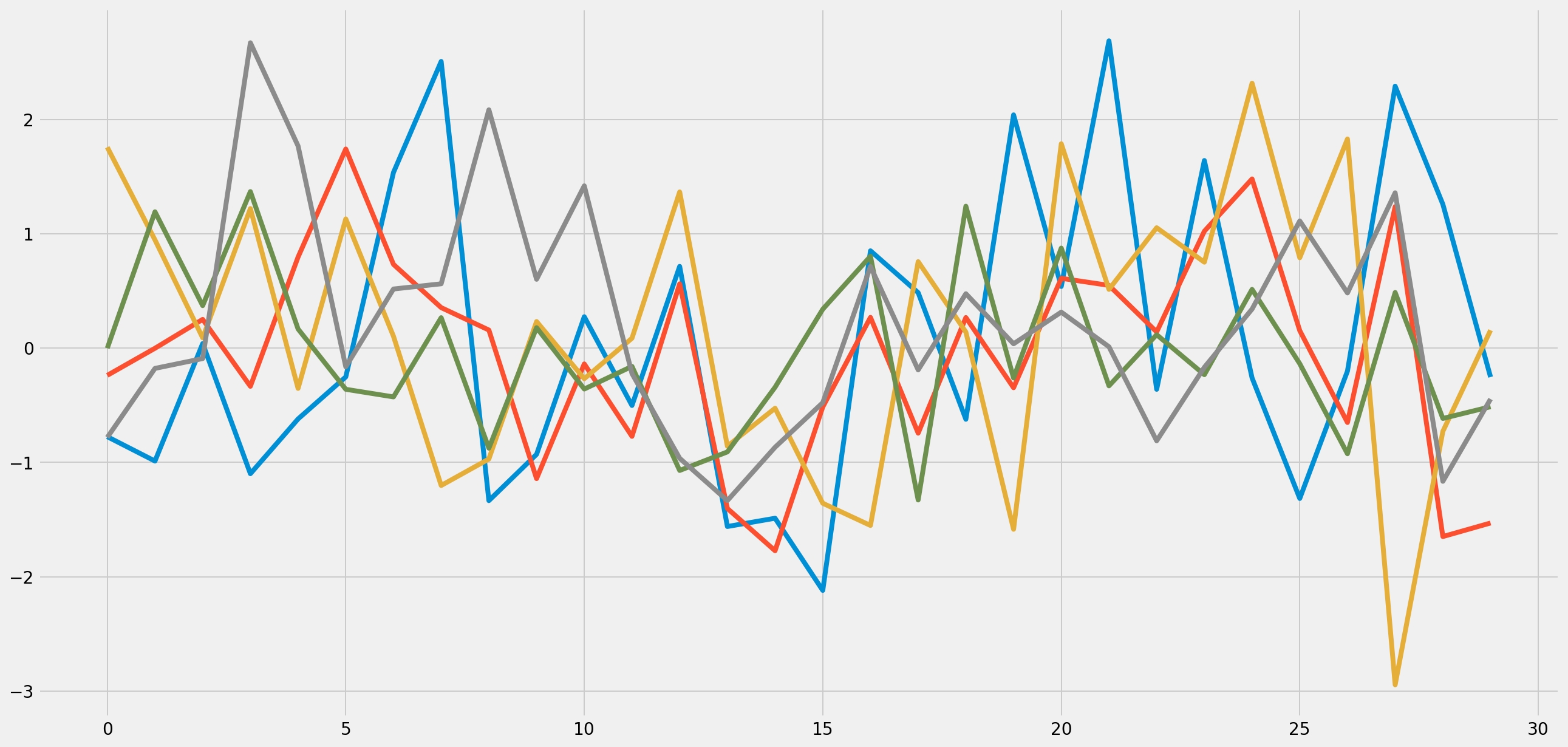generated_arr = generative_model.draw()

plt.style.use("fivethirtyeight")
plt.figure(figsize=(20, 10))
plt.plot(generated_arr)
plt.show()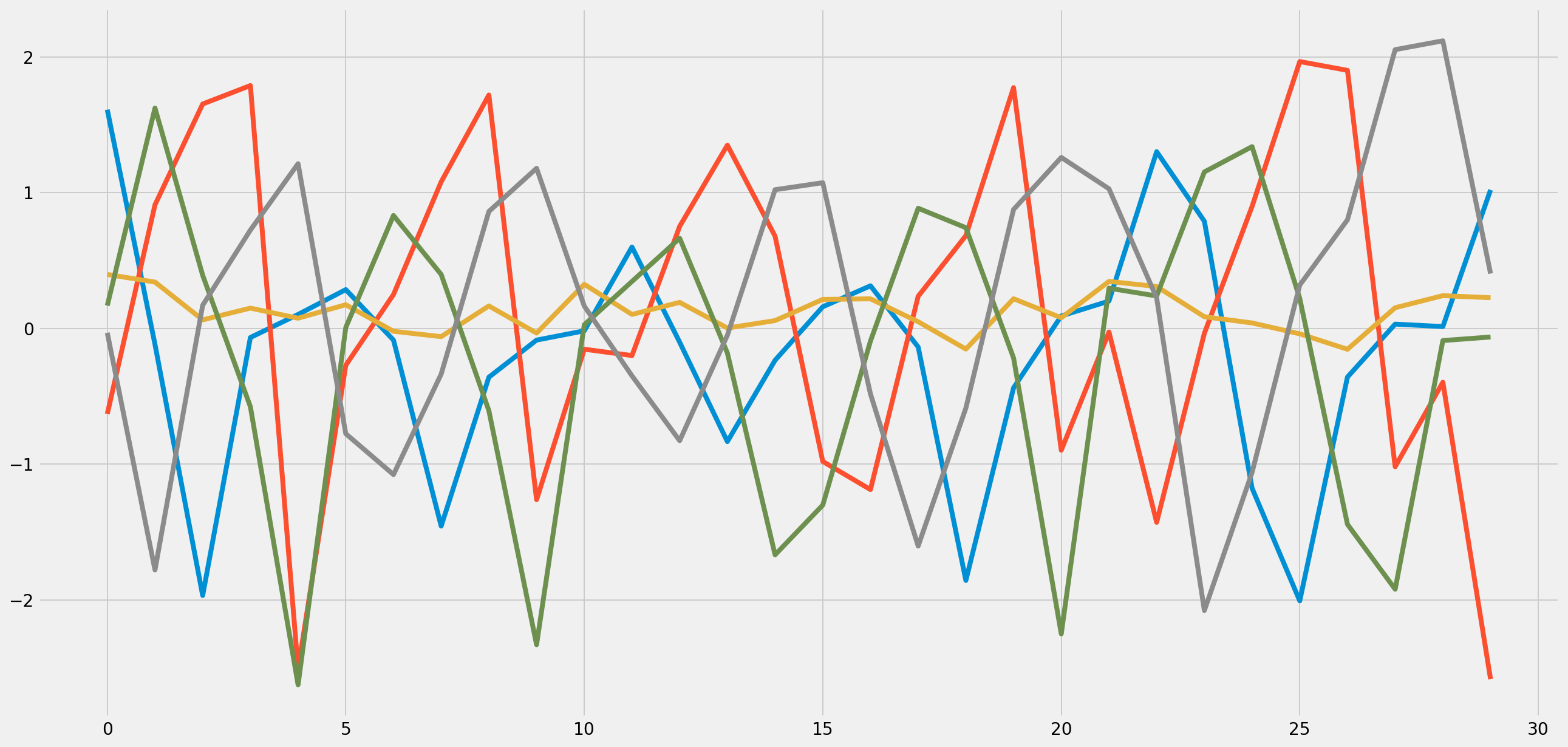Usecase: Generating images by AAEs.¶

In this demonstration, we use image dataset in the Weizmann horse dataset. pydbm library used this dataset to demonstrate for observing reconstruction images by Convolutional Auto-Encoder. and Shape boltzmann machines as follows.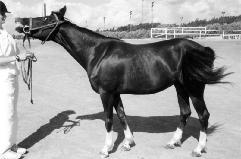Image in the Weizmann horse dataset.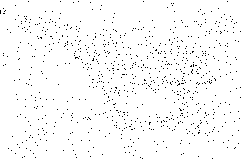Reconstructed image by Shape-BM.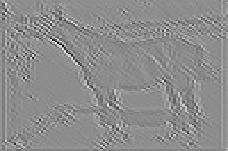Reconstructed image by Convolutional Auto-Encoder.

This library also provides the Convolutional Auto-Encoder, which can be functionally re-used as AutoEncoderModel, loosely coupling with AdversarialAutoEncoders.

Set hyperparameters and directory path that stores your image files.

batch_size = 20
# Width of images.
width = 100
# height of images.
height = 100
# Channel of images.
channel = 1
# Path to your image files.
image_dir = "your/path/to/images/"
# The length of sequneces. If `None`, the objects will ignore sequneces.
seq_len = None
# Gray scale or not.
gray_scale_flag = True
# The tuple of width and height.
wh_size_tuple = (width, height)
# How to normalize pixel values of images.
#   - `z_score`: Z-Score normalization.
#   - `min_max`: Min-max normalization.
#   - `tanh`: Normalization by tanh function.
norm_mode = "z_score"

Import Python modules.

# is-a `TrueSampler`.
from pygan.truesampler.image_true_sampler import ImageTrueSampler
# is-a `NoiseSampler`.
from pygan.noisesampler.image_noise_sampler import ImageNoiseSampler
# is-a `AutoencoderModel`.
from pygan.generativemodel.autoencodermodel.convolutional_auto_encoder import ConvolutionalAutoEncoder as Generator
# is-a `DiscriminativeModel`.
from pygan.discriminativemodel.cnn_model import CNNModel as Discriminator
# Value function.
from pygan.gansvaluefunction.mini_max import MiniMax
# Feature Matching.
from pygan.feature_matching import FeatureMatching

Import pydbm modules.

# Convolution layer.
from pydbm.cnn.layerablecnn.convolution_layer import ConvolutionLayer
# Computation graph in output layer.
from pydbm.synapse.cnn_output_graph import CNNOutputGraph
# Computation graph for convolution layer.
from pydbm.synapse.cnn_graph import CNNGraph
# Logistic Function as activation function.
from pydbm.activation.logistic_function import LogisticFunction
# Tanh Function as activation function.
from pydbm.activation.tanh_function import TanhFunction
# ReLu Function as activation function.
from pydbm.activation.relu_function import ReLuFunction
# SGD optimizer.
from pydbm.optimization.optparams.sgd import SGD
# MSE.
from pydbm.loss.mean_squared_error import MeanSquaredError
# Convolutional Auto-Encoder.
from pydbm.cnn.convolutionalneuralnetwork.convolutional_auto_encoder import ConvolutionalAutoEncoder as CAE
# Deconvolution layer.
from pydbm.cnn.layerablecnn.convolutionlayer.deconvolution_layer import DeconvolutionLayer
# Verification object.
from pydbm.verification.verificate_function_approximation import VerificateFunctionApproximation

Setup TrueSampler.

true_sampler = ImageTrueSampler(
batch_size=batch_size,
image_dir=image_dir,
seq_len=seq_len,
gray_scale_flag=gray_scale_flag,
wh_size_tuple=wh_size_tuple,
norm_mode=norm_mode
)

Setup NoiseSampler and AutoEncoderModel.

noise_sampler = ImageNoiseSampler(
batch_size,
image_dir,
seq_len=seq_len,
gray_scale_flag=gray_scale_flag,
wh_size_tuple=wh_size_tuple,
norm_mode=norm_mode
)

if gray_scale_flag is True:
channel = 1
else:
channel = 3
scale = 0.1

conv1 = ConvolutionLayer(
CNNGraph(
activation_function=TanhFunction(),
# The number of filters.
filter_num=batch_size,
channel=channel,
# Kernel size.
kernel_size=3,
scale=scale,
# The number of strides.
stride=1,
# The number of zero-padding.
)
)

conv2 = ConvolutionLayer(
CNNGraph(
activation_function=TanhFunction(),
filter_num=batch_size,
channel=batch_size,
kernel_size=3,
scale=scale,
stride=1,
)
)

deconvolution_layer_list = [
DeconvolutionLayer(
CNNGraph(
activation_function=TanhFunction(),
filter_num=batch_size,
channel=channel,
kernel_size=5,
scale=scale,
stride=1,
)
)
]

# The probability of dropout.
opt_params.dropout_rate = 0.0

convolutional_auto_encoder = CAE(
layerable_cnn_list=[
conv1,
conv2
],
epochs=100,
batch_size=batch_size,
learning_rate=1e-05,
# # Attenuate the `learning_rate` by a factor of this value every `attenuate_epoch`.
learning_attenuate_rate=0.1,
# # Attenuate the `learning_rate` by a factor of `learning_attenuate_rate` every `attenuate_epoch`.
attenuate_epoch=25,
computable_loss=MeanSquaredError(),
opt_params=opt_params,
verificatable_result=VerificateFunctionApproximation(),
# # Size of Test data set. If this value is `0`, the validation will not be executed.
test_size_rate=0.3,
# Tolerance for the optimization.
# When the loss or score is not improving by at least tol
# for two consecutive iterations, convergence is considered
# to be reached and training stops.
tol=1e-15
)

generator = Generator(
batch_size=batch_size,
learning_rate=1e-05,
convolutional_auto_encoder=convolutional_auto_encoder,
deconvolution_layer_list=deconvolution_layer_list,
gray_scale_flag=gray_scale_flag
)
generator.noise_sampler = noise_sampler

Setup DiscriminativeModel.

convD = ConvolutionLayer(
CNNGraph(
activation_function=TanhFunction(),
filter_num=batch_size,
channel=channel,
kernel_size=3,
scale=0.001,
stride=3,
)
)

layerable_cnn_list=[
convD
]

opt_params.dropout_rate = 0.0

cnn_output_graph = CNNOutputGraph(
# The number of units in hidden layer.
hidden_dim=23120,
# The number of units in output layer.
output_dim=1,
activating_function=LogisticFunction(),
scale=0.01
)

discriminator = Discriminator(
batch_size=batch_size,
layerable_cnn_list=layerable_cnn_list,
cnn_output_graph=cnn_output_graph,
learning_rate=1e-05,
opt_params=opt_params
)

Setup AAEs framework.

gans_value_function=MiniMax(),
feature_matching=FeatureMatching(
# Weight for results of standard feature matching.
lambda1=0.01,
# Weight for results of difference between generated data points and true samples.
lambda2=0.99
)
)

Start pre-training.

generator.pre_learn(true_sampler=true_sampler, epochs=1000)

Start training.

generator, discriminator = AAE.train(
true_sampler=true_sampler,
generative_model=generator,
discriminative_model=discriminator,
iter_n=1000,
k_step=5
)

Visualization.¶

Check the rewards or losses.

Result of pre-training.¶
plt.figure(figsize=(20, 10))
plt.title("The reconstruction errors.")
plt.plot(generator.pre_loss_arr)
plt.show()
plt.close()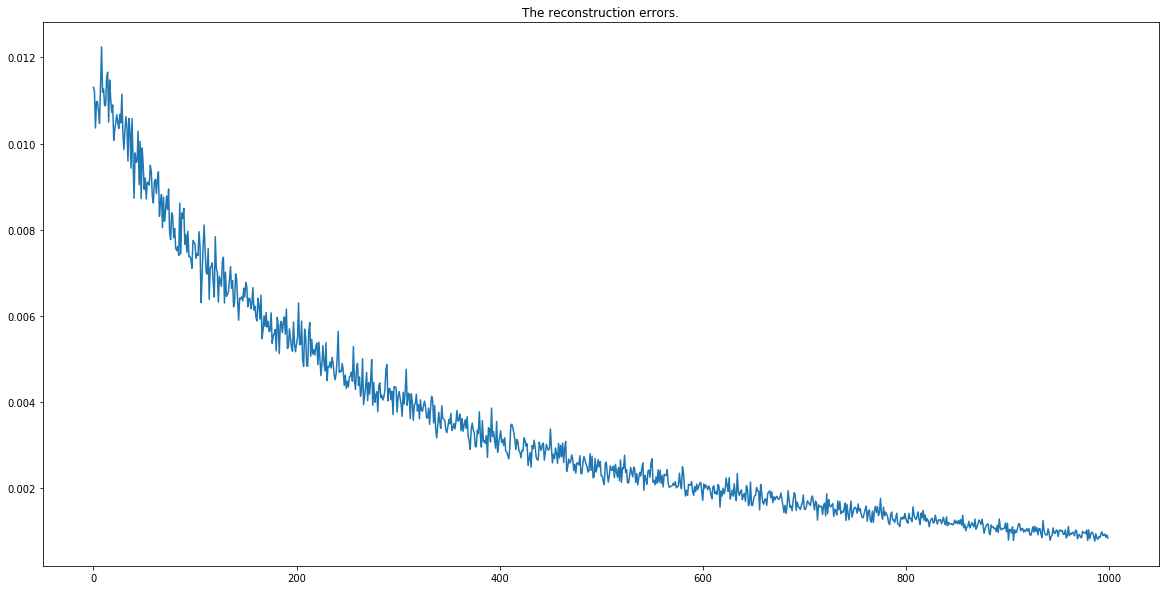Result of training.¶
a_logs_list, d_logs_list, g_logs_list = AAE.extract_logs_tuple()

a_logs_list is a list of the reconstruction errors.

Visualize the values of a_logs_list.

import matplotlib.pyplot as plt
import seaborn as sns
%config InlineBackend.figure_format = "retina"
plt.figure(figsize=(20, 10))
plt.title("The reconstruction errors.")
plt.plot(a_logs_list)
plt.show()
plt.close()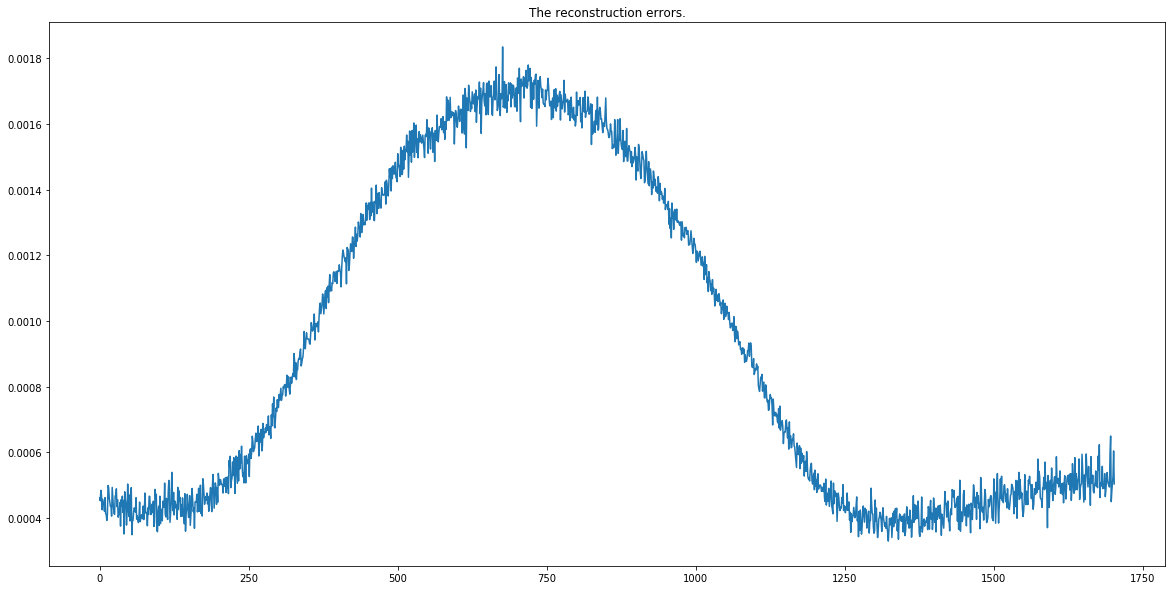The error is not decreasing in steps toward the lower side. Initially, the error is monotonically increased probably due to the side effects of GeneratorModel and DiscriminativeModel learning in GANs framework. However, as learning as an Auto-Encoder progresses gradually in AAEs framework, it converges after showing the tendency of the monotonous phenomenon.

Visualize the values of d_logs_list.

import matplotlib.pyplot as plt
import seaborn as sns
%config InlineBackend.figure_format = "retina"
plt.style.use("fivethirtyeight")
plt.figure(figsize=(20, 10))
plt.plot(d_logs_list)
plt.xlabel("Epochs")
plt.ylabel("Loss")
plt.show()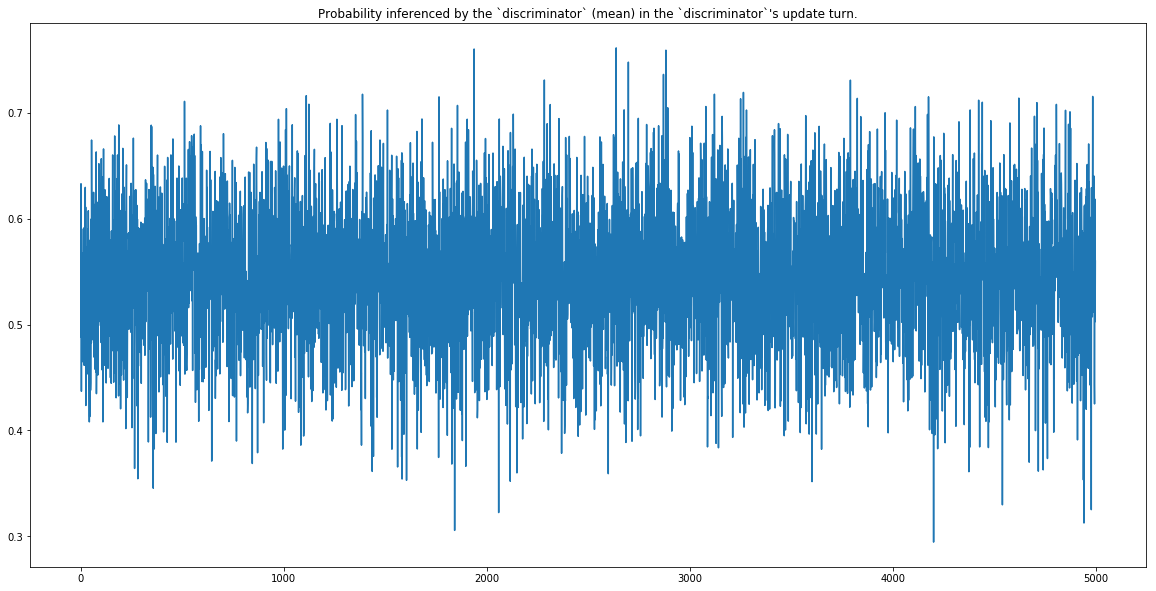Similarly, visualize the values of g_logs_list.

plt.figure(figsize=(20, 10))
plt.plot(g_logs_list)
plt.xlabel("Epochs")
plt.ylabel("Loss")
plt.show()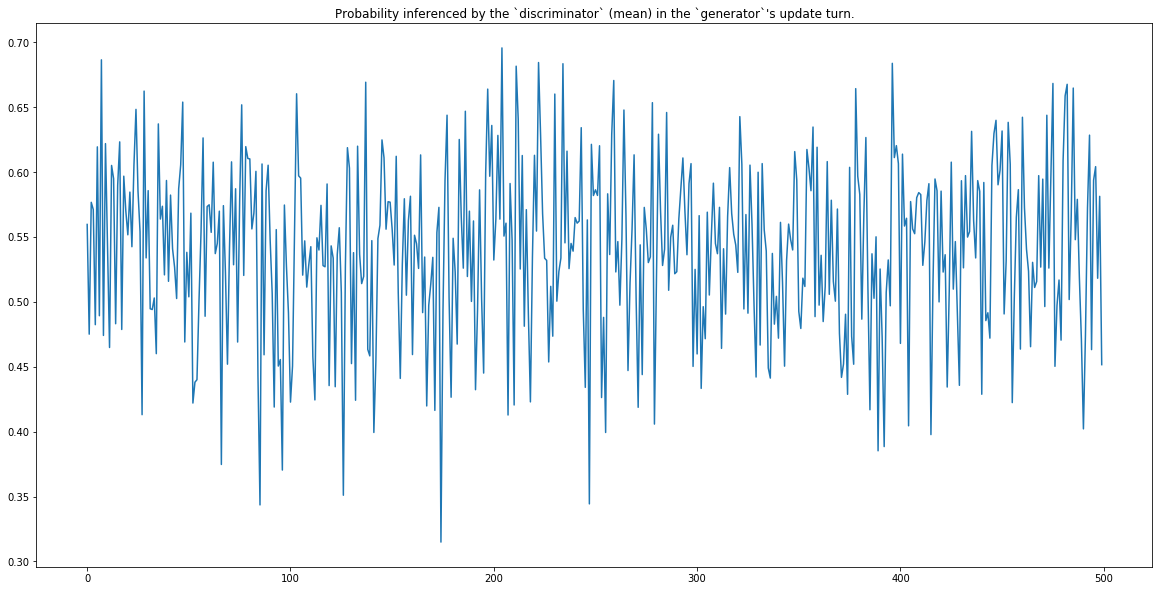As the training progresses, the values are close to not 0.5 but about 0.55.

Apparently it was not perfect. But we can take heart from the generated images.

Generation.¶

Let’s define a helper function for plotting.

def plot(arr):
'''
Plot three gray scaled images.

Args:
arr:    mini-batch data.

'''
for i in range(3):
plt.imshow(arr[i, 0], cmap="gray");
plt.show()
plt.close()

Draw from a true distribution of images and input it to plot function.

arr = true_sampler.draw()
plot(arr)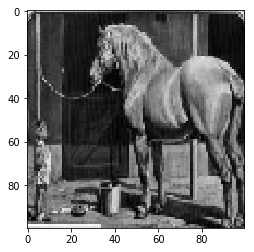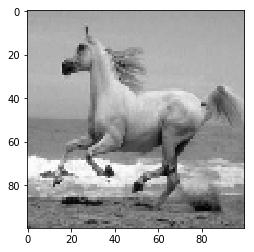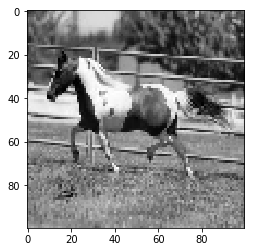Input the generated np.ndarray to plot function.

arr = generator.draw()
plot(arr)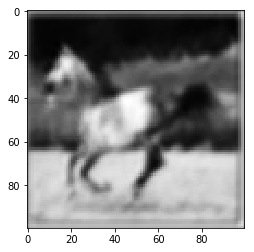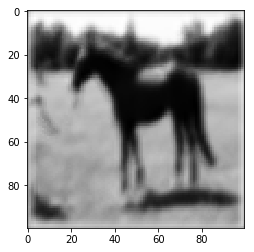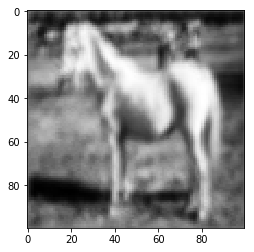Next, observe the true images and reconstructed images.

observed_arr = generator.noise_sampler.generate()
decoded_arr = generator.inference(observed_arr)
plot(observed_arr)
plot(decoded_arr)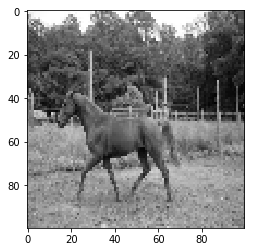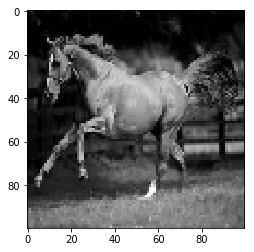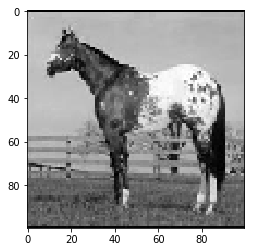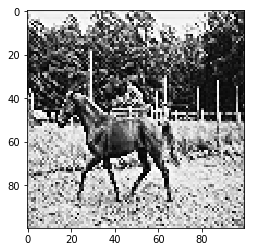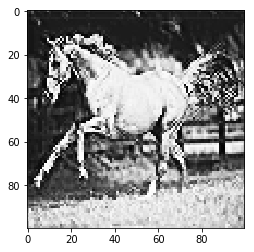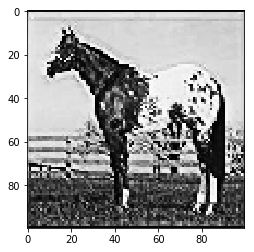About the progress of learning¶

Just observing the results of learning does not tell how learning of each model is progressing. In the following, the progress of each learning step is confirmed from the generated images and the reconstructed images.

Generated images in 500 step.¶
arr = generator.draw()
plot(arr)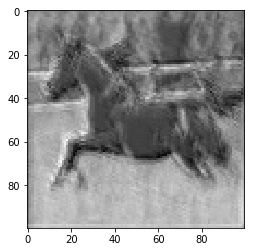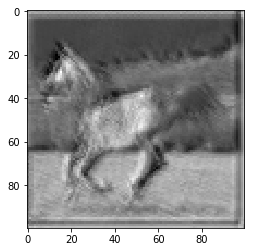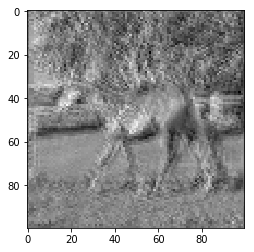Generated images in 1000 step.¶
arr = generator.draw()
plot(arr)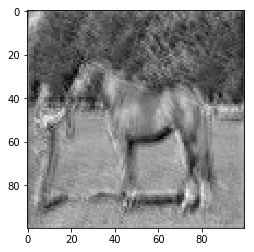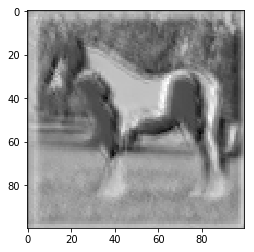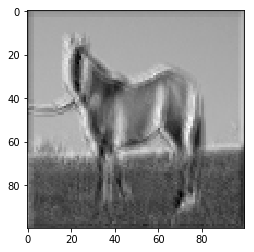Generated images in 1500 step.¶
arr = generator.draw()
plot(arr)Reconstructed images in 500 step.¶
observed_arr = generator.noise_sampler.generate()
decoded_arr = generator.inference(observed_arr)
plot(observed_arr)
plot(decoded_arr)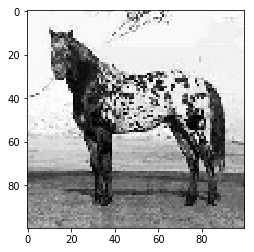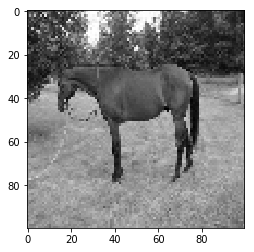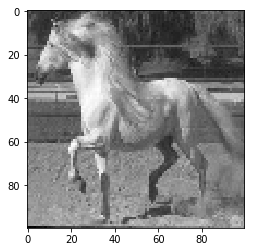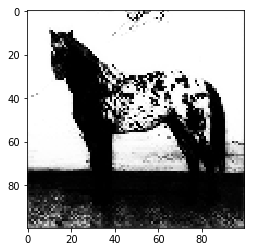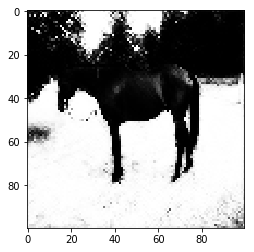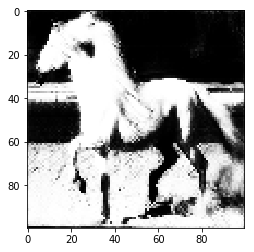Reconstructed images in 1000 step.¶
observed_arr = generator.noise_sampler.generate()
decoded_arr = generator.inference(observed_arr)
plot(observed_arr)
plot(decoded_arr)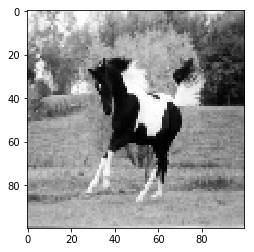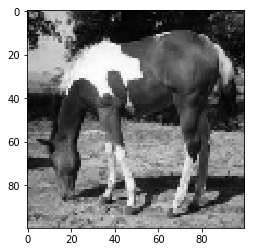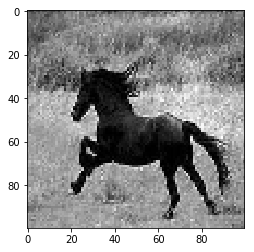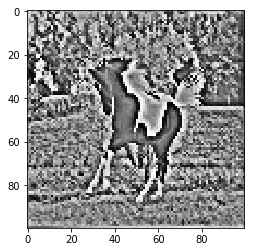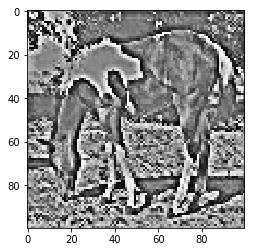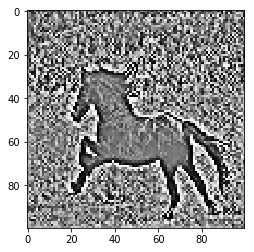Reconstructed images in 1500 step.¶
observed_arr = generator.noise_sampler.generate()
decoded_arr = generator.inference(observed_arr)
plot(observed_arr)
plot(decoded_arr)Author¶

• chimera0(RUM)

Author URI¶

• http://accel-brain.com/